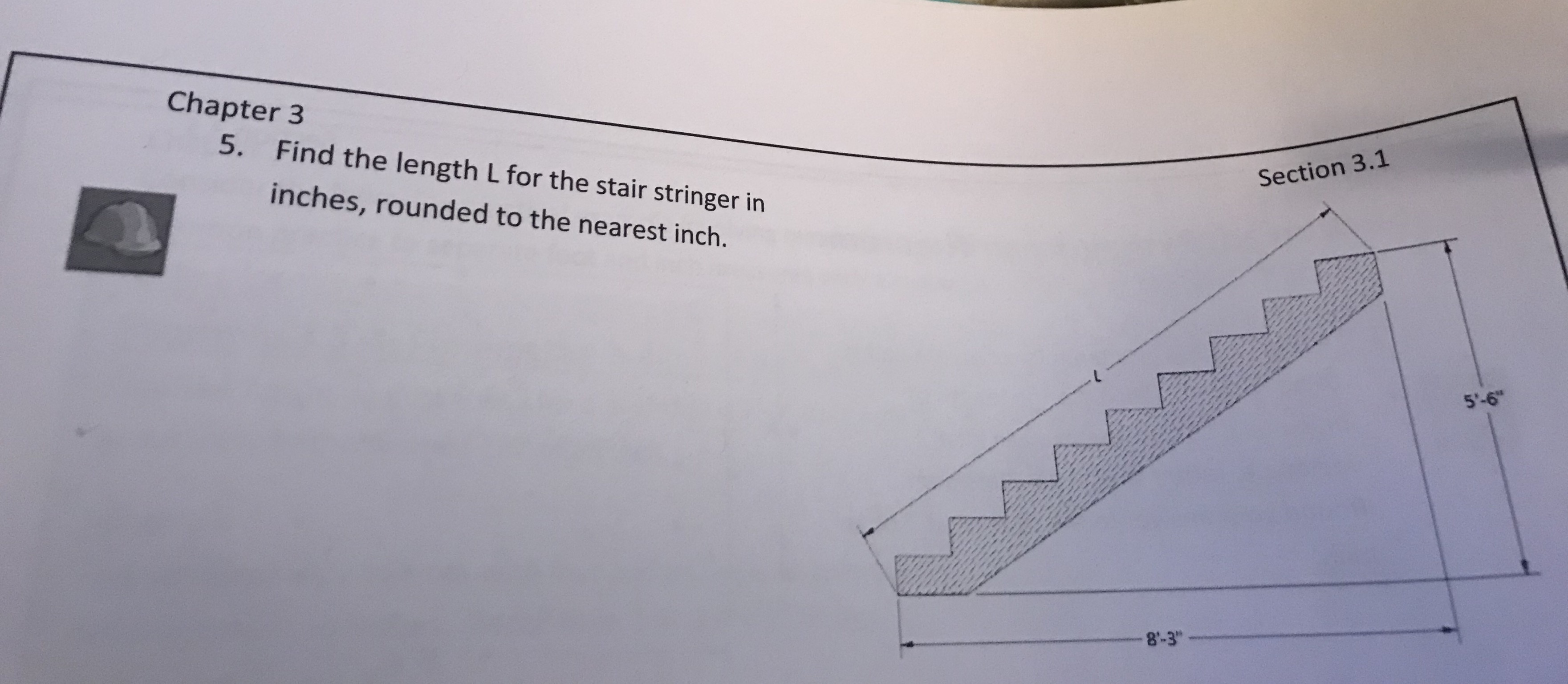# Chapter 3Find the length Lfor the stair stringer ininches, rounded to the nearest inch.Section 3.15'-68-3

Question
36 views

Please write detail explaination, approach and so onhelp_outlineImage TranscriptioncloseChapter 3 Find the length Lfor the stair stringer in inches, rounded to the nearest inch. Section 3.1 5'-6 8-3 fullscreen
check_circle

Step 1

To find the length L for the stair stringer in inches, rounded to the nearest inch.

Consider the stair as a right angled triangle. The given measures will be the base and altitude of the triangle. Where as the unknown L will be the hypotenuse of the triangle. With the help of Pythagoras theorem, the unknown can be found out.

The pythagoras theorem states that: "If a and b be the base and altitude of a right angled triangle. And c be the hypotenuse of the same triangle, then the three sides are connected with an equality: a2+b2=c2."

Step 2

The given base is b = 8'3" = (12*8) + 3 = 99"

The given altitude is a = 5'6" = (12*5) +...

### Want to see the full answer?

See Solution

#### Want to see this answer and more?

Solutions are written by subject experts who are available 24/7. Questions are typically answered within 1 hour.*

See Solution
*Response times may vary by subject and question.
Tagged in

### Geometry